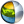Cg 3.1 Toolkit Documentation Cg / Standard Library / reflect

## Name

reflect - returns the reflectiton vector given an incidence vector and a normal vector.

## Synopsis

```float  reflect(float  i, float  n);
float2 reflect(float2 i, float2 n);
float3 reflect(float3 i, float3 n);
float4 reflect(float4 i, float4 n);
```

## Parameters

i
Incidence vector.
n
Normal vector.

## Description

Returns the reflectiton vector given an incidence vector i and a normal vector n. The resulting vector is the identical number of components as the two input vectors.

The normal vector n should be normalized. If n is normalized, the output vector will have the same length as the input incidence vector i.

## Reference Implementation

reflect for float3 vectors could be implemented this way:

```float3 reflect( float3 i, float3 n )
{
return i - 2.0 * n * dot(n,i);
}
```

## Profile Support

reflect is supported in all profiles.

Support in the fp20 is limited.| Cg Toolkit | Download | Release Archive | Profiles | Reference | Books | Discussions |